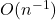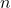# Comparison study on the confidence intervals in linear regression models with restricted parameter space

This paper proposes an empirical likelihood confidence region for the regression coefficients in linear regression models when the regression coefficients are subjected to some equality constraints. The shape of the confidence set does not depend on the re-parametrization of the regression model induced by the equality constraint. It is shown that the asymptotic coverage rate attains the nominal confidence level and the Bartlett correction can successfully reduce the coverage error rate fromto, wheredenotes the sample size. Simulation studies are conducted to evaluate the finite sample performance of the proposed empirical likelihood empirical confidence estimation procedure. Finally, a comparison study is conducted to compare the finite sample performance of the proposed and the classical ellipsoidal confidence sets based on normal theory.